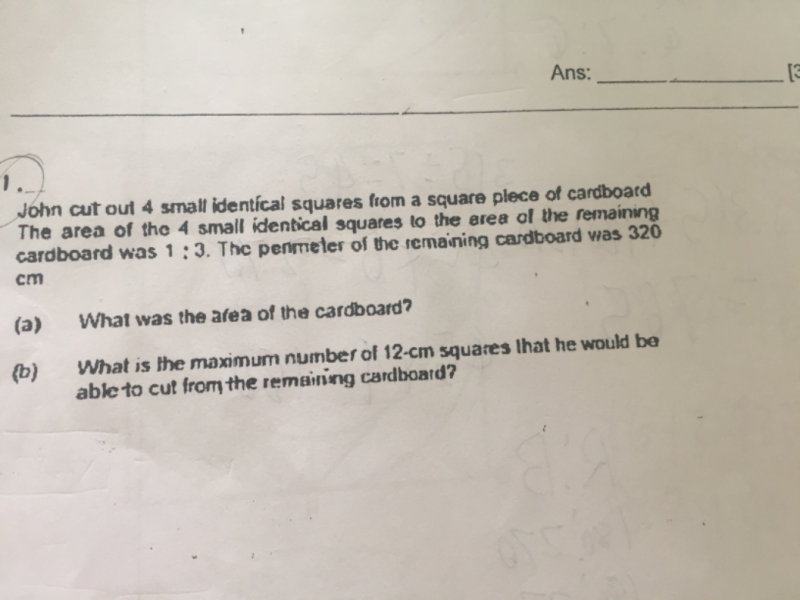# QuestionThis is a Part Whole question.

 Square left Total Quantity 1u 3u 4u Amount 4800 6400

a)

320 ÷ 4 = 80

80 × 80 = 6400

b)

4u = 6400

1u = 6400 ÷ 4 = 1600

3u = 1600 × 3 = 4800

12 × 12 = 144

4800 ÷ 144 =  33.3 ~ 33

2 Replies 0 Likes

Dear Sir,

Can you please tell me how do we do 320 divided by 4.  My confusion is that it is perimeter of remaining carboard.

perimeter of the carboard before squares are cut = 320cm (if the area is 6400 sq cm). After squres are cut, then its perimeter will be less than 320cm right. I’m confused here. Can you please assist

Thanks

0 Replies 0 Likes

Question is not properly set.

Amend the question as follows to remove any ambiguity :

John cut out 4 small identical squares from a square piece of cardboard The area of the 4 small identical squares to the area of the remaining cardboard was 1 : 3.  The perimeter of the remaining cardboard was 320cm.  The length of each small square and the length of the cardboard are whole numbers.

a)What was the maximum possible area of the cardboard ?

b)Using your answer in part (a), what is the maximum number of 12-cm squares that he would be able to cut from the remaining cardboard ?

a) 6400 cm²

b) 29

I have pm you the solution.

cheers.